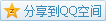# MIT OCW

## 18.02 Multivariable Calculus

### Equations of Planes

#### Expression

The equation of a plane should be in the form of $ax+by+cz=d$.
e.g. Plane through origin with normal vector $\mathbf{N}=(1,5,10)$.
Solution Select a point $P(x,y,z)$ on the plane and we have $\mathbf{OP}\cdot\mathbf{N}=0$, that is $x+5y+10z=0$.

e.g. Plane through $P_0(2,1,-1)$ with normal vector $\mathbf{N}=(1,5,10)$.
It's slightly different but what we will do is almost the same. Select a point $P(x,y,z)$ on the plane and we have $\mathbf{P_0P}\cdot\mathbf{N}=0$, that is $(x-2)+5(y-1)+10(z+1)=0$, simplified as $x+5y+10z=-3$.

Observe these two examples, their coefficients before $x,y,z$ are exactly the components of the normal vector $\mathbf{N}$, the only difference is just the constant. Here the constant can be understood as some offset to origin, since there is no offset when the constant is $0$, namely through the origin.

After we know the fact, we can solve the second example in an easier way. Because we've known the form in the second example is $x+5y+10z=d$, we just plug $P_0(2,1-1)$ into it and solve the value of $d$, finally we get the whole equation.

#### Position

If we know a vector and the equation of a plane, we can judge their relationship by the above trick.
e.g. Given a vector $\mathbf{v}=(1,2,-1)$ and a plane $x+y+3z=5$, what's their relationship? Parallel / Perpendicular / Neither.
Solution The normal vector of the plane is $\mathbf{N}=(1,1,3)$, and we find that $\mathbf{N}\cdot\mathbf{v}=0$. Caution! It DOES NOT mean $\mathbf{v}$ is perpendicular to the plane, instead, what we check is $\mathbf{v}$ and the normal vector $\mathbf{N}$ of the plane. Therefore, it oppositely suggests that the vector $\mathbf{v}$ is parallel to the plane.

### Linear Systems

Think about a $3\times 3$ linear system, for example, \left\{\begin{alignedat}{4} x & {}+{} & & {}{} & z & {}={} & 1 \\ x & {}+{} & y & {} {} & & {}={} & 2 \\ x & {}+{} & 2y & {}+{} & 3z & {}={} & 3\end{alignedat}\right..
These three equations are all planes, and what's the meaning of a solution?
In geometry, we can only find only one solution when the first two planes intersect in a line, and the line intersects with the third plane with a point. To solve this, a good way is to use matrix, a.k.a. $\mathbf{A}\mathbf{X}=\mathbf{B}\Leftrightarrow\mathbf{X}=\mathbf{A}^{-1}\mathbf{B}$.
Anyhow, there exists some weird situtations and the method doesn't work. Generally, the solution of the system have four possibilities:

• No solutions
• One point (unique solution)
• A line (infinite solutions)
• A plane (infinite solutions)

If two planes are parallel, then there exists no solutions at all.
If three planes are all the same, then there exists infinite solutions, namely a plane.
If two planes intersect in a line, and the thrid plane is parallel to the line (not contained), then there exists no solutions.
If two planes intersect in a line, and the thrid plane is contain the line, then there exists infinite solutions, namely a line.

#### Invertible Matrices

What's above method going wrong in algebra? The formula $\mathbf{A}^{-1}=\frac{\operatorname{adj}(\mathbf{A})}{\det(\mathbf{A})}$ is definitely correct, but what will happen if $\det(\mathbf{A})=0$? We say that those matrices whose determinant $\det(\mathbf{A})\neq 0$ are invertible and they have inverse, the others are not invertible and don't have inverse.

#### Homogeneous Systems

A homogeneous system has the form $\mathbf{A}\mathbf{X}=0$，we can find that no matter what $\mathbf{A}$ is, obviously $\mathbf{X}=0$ is always a solution, which is called a trivial solution.
If $\det(\mathbf{A})\neq 0$, $\mathbf{X}=0$ is the unique solution.
If $\det(\mathbf{A})=0$, then $\det(\mathbf{N_1},\mathbf{N_2},\mathbf{N_3})=0$, which means their normal vectors are coplanar. Then there exists nontrivial solutions like $\mathbf{X}=\mathbf{N_1}\times\mathbf{N_2}$.

#### General Cases

For a system $\mathbf{A}\mathbf{X}=\mathbf{B}$

• if $\det(\mathbf{A})\neq 0$, then it has unique solution
• if $\det(\mathbf{A})=0$, then it has either no solutions or infinite solutions.

The more details will be found when trying to solve the system by elimination and substitution, but if we know $\det(\mathbf{A})=0$ and find a solution, then it has to have infinite solutions; and when we get something contradictory, then it turns out that the system has no solutions.